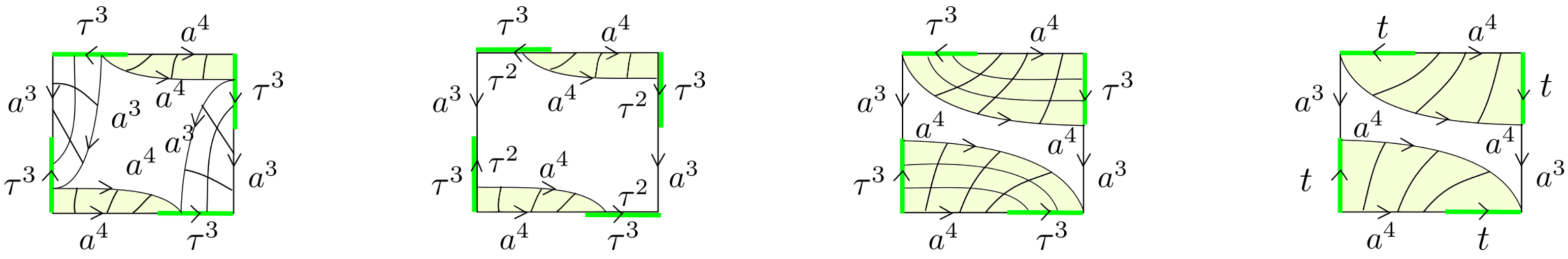Dehn functions of mapping tori of right-angled Artin groups The algebraic mapping torus $M_{\Phi}$ of a group $G$ with an automorphism $\Phi$ is the HNN-extension of $G$ in which conjugation by the stable letter performs $\Phi$. We classify the Dehn functions of $M_{\Phi}$ in terms of $\Phi$ for a number of right-angled Artin groups $G$, including all $3$-generator right-angled Artin groups and $F_k \times F_l$ for all $k,l \geq 2$.